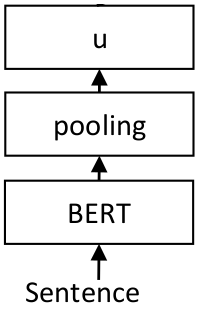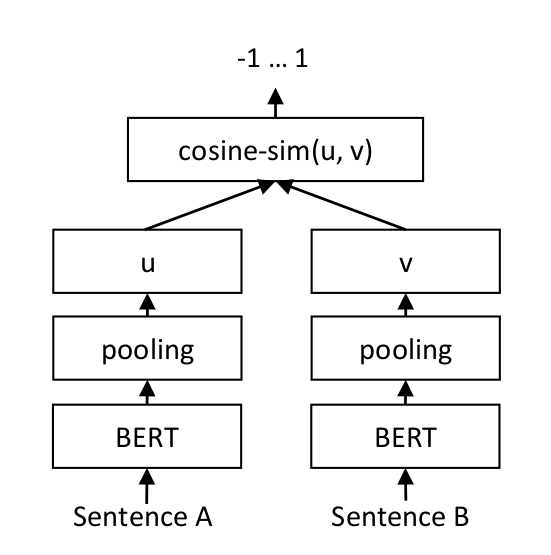# Training Overview¶

Each task is unique, and having sentence / text embeddings tuned for that specific task greatly improves the performance.

SentenceTransformers was designed in such way that fine-tuning your own sentence / text embeddings models is easy. It provides most of the building blocks that you can stick together to tune embeddings for your specific task.

Sadly there is no single training strategy that works for all use-cases. Instead, which training strategy to use greatly depends on your available data and on your target task.

In the Training section, I will discuss the fundamentals of training your own embedding models with SentenceTransformers. In the Training Examples section, I will provide examples how to tune embedding models for common real-world applications.

## Network Architecture¶

For sentence / text embeddings, we want to map a variable length input text to a fixed sized dense vector. The most basic network architecture we can use is the following:We feed the input sentence or text into a transformer network like BERT. BERT produces contextualized word embeddings for all input tokens in our text. As we want a fixed-sized output representation (vector u), we need a pooling layer. Different pooling options are available, the most basic one is mean-pooling: We simply average all contextualized word embeddings BERT is giving us. This gives us a fixed 768 dimensional output vector independent how long our input text was.

The depicted architecture, consisting on a BERT layer and a pooling layer is one final SentenceTransformer model.

## Creating Networks from Scratch¶

In the quick start & usage examples, we used pre-trained SentenceTransformer models that already come with a BERT layer and a pooling layer.

But we can create the networks architectures from scratch by defining the individual layers. For example, the following code would create the depicted network architecture:

```from sentence_transformers import SentenceTransformer, models

word_embedding_model = models.Transformer('bert-base-uncased', max_seq_length=256)
pooling_model = models.Pooling(word_embedding_model.get_word_embedding_dimension())

model = SentenceTransformer(modules=[word_embedding_model, pooling_model])
```

First we define our individual layers, in this case, we define ‘bert-base-uncased’ as the word_embedding_model. We limit that layer to a maximal sequence length of 256, texts longer than that will be truncated. Further, we create a (mean) pooling layer. We create a new SentenceTransformer model by calling `SentenceTransformer(modules=[word_embedding_model, pooling_model])`. For the modules parameter, we pass a list of layers which are executed consecutively. Input text are first passed to the first entry (word_embedding_model). The output is then passed to the second entry (pooling_model), which then returns our sentence embedding.

We can also construct more complex models:

```from sentence_transformers import SentenceTransformer, models
from torch import nn

word_embedding_model = models.Transformer('bert-base-uncased', max_seq_length=256)
pooling_model = models.Pooling(word_embedding_model.get_word_embedding_dimension())
dense_model = models.Dense(in_features=pooling_model.get_sentence_embedding_dimension(), out_features=256, activation_function=nn.Tanh())

model = SentenceTransformer(modules=[word_embedding_model, pooling_model, dense_model])
```

Here, we add a on top of the pooling layer a fully connected dense layer with Tanh activation, which performs a down-project to 256 dimensions. Hence, embeddings by this model will only have 256 instead of 768 dimensions.

For all available building blocks see » Models Package Reference

## Training Data¶

To represent our training data, we use the `InputExample` class to store training examples. As parameters, it accepts texts, which is a list of strings representing our pairs (or triplets). Further, we can also pass a label (either float or int). The following shows a simple example, where we pass text pairs to `InputExample` together with a label indicating the semantic similarity.

```from sentence_transformers import SentenceTransformer, InputExample

model = SentenceTransformer('distilbert-base-nli-mean-tokens')
train_examples = [InputExample(texts=['My first sentence', 'My second sentence'], label=0.8),
InputExample(texts=['Another pair', 'Unrelated sentence'], label=0.3)]
```

We wrap our `train_examples` with the standard PyTorch `DataLoader`, which shuffles our data and produces batches of certain sizes.

## Loss Functions¶

The loss function plays a critical role when fine-tuning the model. It determines how well our embedding model will work for the specific downstream task.

Sadly there is no “one size fits all” loss function. Which loss function is suitable depends on the available training data and on the target task.

To fine-tune our network, we need somehow to tell our network which sentence pairs are similar, and should be close in vector space, and which pairs are dissimilar, and should be far away in vector space.

The most simple way is to have sentence pairs annotated with a score indicating their similarity, e.g. on a scale 0 to 1. We can then train the network with a Siamese Network Architecture (for details see: Sentence-BERT: Sentence Embeddings using Siamese BERT-Networks)For each sentence pair, we pass sentence A and sentence B through our network which yields the embeddings u und v. The similarity of these embeddings is computed using cosine similarity and the result is compared to the gold similarity score. This allows our network to be fine-tuned and to recognize the similarity of sentences.

A minimal example with `CosineSimilarityLoss` is the following:

```from sentence_transformers import SentenceTransformer, InputExample, losses

#Define the model. Either from scratch of by loading a pre-trained model
model = SentenceTransformer('distilbert-base-nli-mean-tokens')

#Define your train examples. You need more than just two examples...
train_examples = [InputExample(texts=['My first sentence', 'My second sentence'], label=0.8),
InputExample(texts=['Another pair', 'Unrelated sentence'], label=0.3)]

train_loss = losses.CosineSimilarityLoss(model)

#Tune the model
```

We tune the model by calling model.fit(). We pass a list of `train_objectives`, which constist of tuples `(dataloader, loss_function)`. We can pass more than one tuple in order to perform multi-task learning on several datasets with different loss functions.

The `fit` method accepts the following parameter:

class `sentence_transformers.``SentenceTransformer`(model_name_or_path: Optional[str] = None, modules: Optional[Iterable[torch.nn.modules.module.Module]] = None, device: Optional[str] = None, cache_folder: Optional[str] = None)

Loads or create a SentenceTransformer model, that can be used to map sentences / text to embeddings.

Parameters
• model_name_or_path – If it is a filepath on disc, it loads the model from that path. If it is not a path, it first tries to download a pre-trained SentenceTransformer model. If that fails, tries to construct a model from Huggingface models repository with that name.

• modules – This parameter can be used to create custom SentenceTransformer models from scratch.

• device – Device (like ‘cuda’ / ‘cpu’) that should be used for computation. If None, checks if a GPU can be used.

• cache_folder – Path to store models

Initializes internal Module state, shared by both nn.Module and ScriptModule.

`fit`(train_objectives: Iterable[Tuple[torch.utils.data.dataloader.DataLoader, torch.nn.modules.module.Module]], evaluator: sentence_transformers.evaluation.SentenceEvaluator.SentenceEvaluator = None, epochs: int = 1, steps_per_epoch=None, scheduler: str = 'WarmupLinear', warmup_steps: int = 10000, optimizer_class: Type[torch.optim.optimizer.Optimizer] = <class 'transformers.optimization.AdamW'>, optimizer_params: Dict[str, object] = {'lr': 2e-05}, weight_decay: float = 0.01, evaluation_steps: int = 0, output_path: str = None, save_best_model: bool = True, max_grad_norm: float = 1, use_amp: bool = False, callback: Callable[[float, int, int], None] = None, show_progress_bar: bool = True, checkpoint_path: str = None, checkpoint_save_steps: int = 500, checkpoint_save_total_limit: int = 0)

Train the model with the given training objective Each training objective is sampled in turn for one batch. We sample only as many batches from each objective as there are in the smallest one to make sure of equal training with each dataset.

Parameters
• train_objectives – Tuples of (DataLoader, LossFunction). Pass more than one for multi-task learning

• evaluator – An evaluator (sentence_transformers.evaluation) evaluates the model performance during training on held-out dev data. It is used to determine the best model that is saved to disc.

• epochs – Number of epochs for training

• steps_per_epoch – Number of training steps per epoch. If set to None (default), one epoch is equal the DataLoader size from train_objectives.

• scheduler – Learning rate scheduler. Available schedulers: constantlr, warmupconstant, warmuplinear, warmupcosine, warmupcosinewithhardrestarts

• warmup_steps – Behavior depends on the scheduler. For WarmupLinear (default), the learning rate is increased from o up to the maximal learning rate. After these many training steps, the learning rate is decreased linearly back to zero.

• optimizer_class – Optimizer

• optimizer_params – Optimizer parameters

• weight_decay – Weight decay for model parameters

• evaluation_steps – If > 0, evaluate the model using evaluator after each number of training steps

• output_path – Storage path for the model and evaluation files

• save_best_model – If true, the best model (according to evaluator) is stored at output_path

• use_amp – Use Automatic Mixed Precision (AMP). Only for Pytorch >= 1.6.0

• callback – Callback function that is invoked after each evaluation. It must accept the following three parameters in this order: score, epoch, steps

• show_progress_bar – If True, output a tqdm progress bar

• checkpoint_path – Folder to save checkpoints during training

• checkpoint_save_steps – Will save a checkpoint after so many steps

• checkpoint_save_total_limit – Total number of checkpoints to store

## Evaluators¶

During training, we usually want to measure the performance to see if the performance improves. For this, the sentence_transformers.evaluation package exists. It contains various evaluators which we can pass to the `fit`-method. These evaluators are run periodically during training. Further, they return a score and only the model with the highest score will be stored on disc.

The usage is simple:

```from sentence_transformers import evaluation
sentences1 = ['This list contains the first column', 'With your sentences', 'You want your model to evaluate on']
sentences2 = ['Sentences contains the other column', 'The evaluator matches sentences1[i] with sentences2[i]', 'Compute the cosine similarity and compares it to scores[i]']
scores = [0.3, 0.6, 0.2]

evaluator = evaluation.EmbeddingSimilarityEvaluator(sentences1, sentences2, scores)

model.fit(train_objectives=[(train_dataloader, train_loss)], epochs=1, warmup_steps=100, evaluator=evaluator, evaluation_steps=500)
```

### Continue Training on Other Data¶

training_stsbenchmark_continue_training.py shows an example where training on a fine-tuned model is continued. In that example, we use a sentence transformer model that was first fine-tuned on the NLI dataset and then continue training on the training data from the STS benchmark.

First, we load a pre-trained model from the server:

```model = SentenceTransformer('bert-base-nli-mean-tokens')
```

The next steps are as before. We specify training and dev data:

```train_dataloader = DataLoader(train_samples, shuffle=True, batch_size=train_batch_size)
train_loss = losses.CosineSimilarityLoss(model=model)

```

In that example, we use CosineSimilarityLoss, which computes the cosine similarity between two sentences and compares this score with a provided gold similarity score.

Then we can train as before:

```model.fit(train_objectives=[(train_dataloader, train_loss)],
evaluator=evaluator,
epochs=num_epochs,
evaluation_steps=1000,
warmup_steps=warmup_steps,
output_path=model_save_path)
```

```model = SentenceTransformer('./my/path/to/model/')
```

Note: It is important that a / or \ is the path, otherwise, it is not recognized as a path.

You can also host the training output on a server and download it:

```model = SentenceTransformer('http://www.server.com/path/to/model/my_model.zip')
```

With the first call, the model is downloaded and stored in the local torch cache-folder (`~/.cache/torch/sentence_transformers`). In order to work, you must zip all files and subfolders of your model.

This code allows multi-task learning with training data from different datasets and with different loss-functions. For an example, see training_multi-task.py.

Depending on the task, you might want to special tokens to the tokenizer and the Transformer model. You can use the following code-snippet to achieve this:

```from sentence_transformers import SentenceTransformer, models
word_embedding_model = models.Transformer('bert-base-uncased')

tokens = ["[DOC]", "[QRY]"]
word_embedding_model.auto_model.resize_token_embeddings(len(word_embedding_model.tokenizer))

pooling_model = models.Pooling(word_embedding_model.get_word_embedding_dimension())
model = SentenceTransformer(modules=[word_embedding_model, pooling_model])
```

If you want to extend the vocabulary for an existent SentenceTransformer model, you can use the following code:

```from sentence_transformers import SentenceTransformer, models
model = SentenceTransformer('paraphrase-distilroberta-base-v1')
word_embedding_model = model._first_module()

tokens = ["[DOC]", "[QRY]"]
In the above example, the two new tokens `[DOC]` and `[QRY]` are added to the model. Their respective word embeddings are intialized randomly. It is advisable to then fine-tune the model on your downstream task.